Download Presentation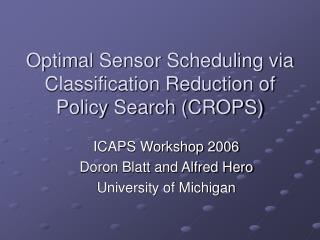Optimal Sensor Scheduling via Classification Reduction of Policy Search (CROPS)

# Optimal Sensor Scheduling via Classification Reduction of Policy Search (CROPS) - PowerPoint PPT Presentation

Optimal Sensor Scheduling via Classification Reduction of Policy Search (CROPS). ICAPS Workshop 2006 Doron Blatt and Alfred Hero University of Michigan. Motivating Example: Landmine Detection. EMI. GPR. Seismic.I am the owner, or an agent authorized to act on behalf of the owner, of the copyrighted work described.
Download Presentation## Optimal Sensor Scheduling via Classification Reduction of Policy Search (CROPS)

An Image/Link below is provided (as is) to download presentation

Download Policy: Content on the Website is provided to you AS IS for your information and personal use and may not be sold / licensed / shared on other websites without getting consent from its author.While downloading, if for some reason you are not able to download a presentation, the publisher may have deleted the file from their server.

- - - - - - - - - - - - - - - - - - - - - - - - - - E N D - - - - - - - - - - - - - - - - - - - - - - - - - -
Presentation Transcript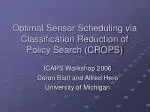### Optimal Sensor Scheduling via Classification Reduction ofPolicy Search (CROPS)

ICAPS Workshop 2006

Doron Blatt and Alfred Hero

University of Michigan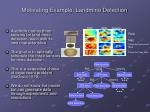Motivating Example: Landmine Detection

EMI

GPR

Seismic

• A vehicle carries three sensors for land-mine detection, each with its own characteristics.
• The goal is to optimally schedule the three sensors for mine detection.
• This is a sequential choice of experiment problem (DeGroot 1970).
• We do not know the model but can generate data through experiments and simulations.

Rock

Nail

Plastic Anti-personnel

Mine

Plastic Anti-tank Mine

New location

EMI

Seismic

GPR

EMI data

GPR data

Seismic data

EMI

Seismic

EMI data

Final detection

Seismic data

Seismic data

Final detection

Final detection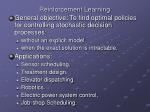Reinforcement Learning
• General objective: To find optimal policies for controlling stochastic decision processes:
• without an explicit model.
• when the exact solution is intractable.
• Applications:
• Sensor scheduling.
• Treatment design.
• Elevator dispatching.
• Robotics.
• Electric power system control.
• Job-shop Scheduling.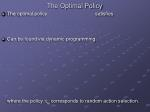The Optimal Policy
• The optimal policy satisfies
• Can be found via dynamic programming:

where the policy qt corresponds to random action selection.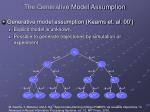O0

a0=0

a0=1

O10

O11

a1=0

a1=1

a1=0

a1=1

O200

O201

O210

O211

a2=0

a2=1

a2=0

a2=1

a2=0

a2=1

a2=0

a2=1

O3000

O3001

O3010

O3011

O3100

O3101

O3110

O3111

The Generative Model Assumption
• Generative model assumption (Kearns et. al. 00’)
• Explicit model is unknown.
• Possible to generate trajectories by simulation or experiment

M. Kearns, Y. Mansour, and A. Ng, “Approximate planning in large POMDPs via reusable trajectories,” in Advances in Neural Information Processing Systems, vol. 12. MIT Press, 2000.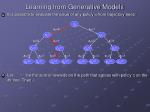Learning from Generative Models

• It is possible to evaluate the value of any policy  from trajectory trees:
• Let be the sum of rewards on the path that agrees with policy  on the ith tree. Then,

O0

a0=0

a0=1

O10

O11

a1=0

a1=1

a1=0

a1=1

O200

O201

O210

O211

a2=0

a2=1

a2=0

a2=1

a2=0

a2=1

a2=0

a2=1

O3000

O3001

O3010

O3011

O3100

O3101

O3110

O3111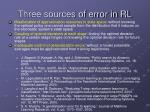Three sources of error in RL
• Misallocation of approximation resources to state space: without knowing the optimal policy one cannot sample from the distribution that it induces on the stochastic system’s state space
• Coupling of optimal decisions at each stage: finding the optimal decision rule at a certain stage hinges on knowing the optimal decision rule for future stages
• Inadequate control of generalization errors: without a model ensemble averages must be approximated from training trajectories
• J. Bagnell, S. Kakade, A. Ng, and J. Schneider, “Policy search by dynamic programming,” in Advances in Neural Information Processing Systems, vol. 16. 2003.
• A. Fern, S. Yoon, and R. Givan, “Approximate policy iteration with a policy language bias,” in Advances in Neural Information Processing Systems, vol. 16, 2003.
• M. Lagoudakis and R. Parr, “Reinforcement learning as classification: Leveraging modern classifiers,” in Proceedings of the Twentieth International Conference on Machine Learning, 2003.
• J. Langford and B. Zadrozny, “Reducing T-step reinforcement learning to classification,” http://hunch.net/∼jl/projects/reductions/reductions.html, 2003.
• M. Kearns, Y. Mansour, and A. Ng, “Approximate planning in large POMDPs via reusable trajectories,” in Advances in Neural Information Processing Systems, vol. 12. MIT Press, 2000.
• S. A. Murphy, “A generalization error for Q-learning,” Journal of Machine Learning Research, vol. 6, pp. 1073–1097, 2005.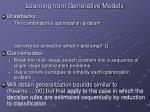Learning from Generative Models
• Drawbacks:
• The combinatorial optimization problem:

can only be solved for small n and small .

• Our remedies:
• Break the multi-stage search problem into a sequence of single-stage optimization problems.
• Use a convex surrogate to simplify each optimization problem.
• Will obtain generalization bounds similar to (Kearns…,’00) but that apply to the case in which the decision rules are estimated sequentially by reduction to classification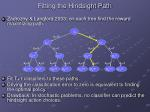Fitting the Hindsight Path
• Zadrozny & Langford 2003: on each tree find the reward maximizing path.
• Fit T+1 classifiers to these paths.
• Driving the classification error to zero is equivalent to finding the optimal policy.
• Drawback: In stochastic problems, no classifier can predict the hindsight action choices.

O0

a0=0

a0=1

O10

O11

a1=0

a1=1

a1=0

a1=1

O200

O201

O210

O211

a2=0

a2=1

a2=0

a2=1

a2=0

a2=1

a2=0

a2=1

O3000

O3001

O3010

O3011

O3100

O3101

O3110

O3111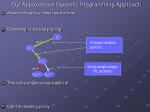Our Approximate Dynamic Programming Approach
• Assume the policy class has the form:
• Estimating T via tree pruning:
• This is the empirical equivalent of:
• Call the resulting policy

O0

a0=0

Choose random actions

O10

a1=1

O201

Solve single-stage

RL problem

a2=0

a2=1

O3010

O3011Our Approximate Dynamic Programming Approach

O0

• Estimating T-1 given via tree pruning:
• This is the empirical equivalent of:

a0=0

Choose random actions

O10

Solve single-stage RL problem

a1=0

a1=1

O200

O201

Propagate rewards according to

a2=0

a2=1

O3000

O3011Our Approximate Dynamic Programming Approach

Propagate rewards according to

• Estimating T-2=0 given and via tree pruning:
• This is the empirical equivalent of:

O0

Solve single-stage RL problem

a0=0

a0=1

O10

O11

a1=1

a1=0

O201

O210

a2=1

a2=1

O3011

O3101O0

a0=-1

a0=1

O1-1

O11

Reduction to Weighted Classification
• Our approximate dynamic programming algorithm converts the multi-stage optimization problem into a sequence of single-stage optimization problems.
• Unfortunately each sequence is still a combinatorial optimization problem.
• Our solution: reduce this to learning classifiers with convex surrogate.
• This classification reduction is different from previous work
• Consider a single-stage RL problem:
• Consider a class of real valued functions
• Each induces a policy:
• We would like to maximizeReduction to Weighted Classification
• Note that
• Therefore, solving a single stage RL problem is equivalent to:

whereReduction to Weighted Classification
• It is often much easier to solvewhere  is a convex function.
• For example:
• In neural network training  is the truncated quadratic loss.
• In boosting is the exponential loss.
• In support vector machines is the hinge loss.
• In logistic regression is the scaled deviance.
• The effect of introducing  is well understood for the classification problem and the results can be applied to the single-stage RL problem as well.Reduction to Weighted ClassificationMulti-Stage Problem
• Let be the policy estimated by the approximate dynamic programming algorithm, where each single-stage RL problem is solved via  minimization.
• Theorem 2: Assume P-dim( ) = dt, t=0, …, T. Then, with probability greater than 1-, over the set of trajectory trees,for n satisfying
• Proof uses recent results in P. L. Bartlett, M. I. Jordan, and J. D. McAulie, “Convexity, classification, and risk bounds,” Journal of the American Statistical Association, vol. 101, no. 473, pp. 138–156, March 2006.
• Tighter than analogous Q-learning bound (Murphy:JMLR2005).Application to Landmine Sensor Scheduling

EMI

GPR

Seismic

• A sand box experiment was conducted by Jay Marble to extract features of the three sensors for different types of land-mines and clutter.
• Based on the results the sensors’ outputs were simulated as a Gaussian mixture.
• Feed forward neural networks were trained to perform both the classification task and the weighted classification talks.
• Performance where evaluated on a separate data set.

Rock

Nail

Plastic Anti-personnel

Mine

Plastic Anti-tank Mine

New location

EMI

Seismic

GPR

EMI data

GPR data

Seismic data

EMI

Seismic

EMI data

Final detection

Seismic data

Seismic data

Final detection

Final detectionReinforcement Learning for Sensor Scheduling Weighted Classification Reduction

Performance obtained by randomized sensor allocation

+

+

+

+

Increasing sensor deployment cost

+

+

Performance obtained by optimal sensor scheduling

+

Always deploy three sensors

+

Always deploy best of two sensors: GRP + Seismic

Always deploy best single sensor: EMIOptimal Policy for Mean States

Policy for specific scenarios:

Optimal sequence for mean state

2

3

D

2

1

D

2

3

D

213

D

2

3

D

2

3

D

2

3

D

2

3

DApplication to waveform selection: Landsat MSS Experiment
• Data consists of 4435 training cases and 2000 test cases.
• Each case is a 3x3x4 image stack in 36 dimensions having 1 class attribute
• (1) Red soil, (2) Cotton, (3)Vegetation stubble, (4) Gray soil, (5) Damp gray soil, (6)Very damp gray soilWaveform Scheduling: CROPS

Bands (1,4)

• For each image location we adopt two stage policy to classify its label:
• Select one of 6 possible pairs of 4 MSS bands for initial illumination
• Based on initial measurement either:
• Make final decision on terrain class and stop
• Illuminate with remaining two MSS bands and make final decision
• Reward is average probability of correct decision minus stopping time (energy)

New location

Bands (1,2)

Bands (1,3)

Bands (2,3)

Bands (2,4)

Bands (3,4)

Classify

Bands (1,4)

Reward=I(correct)

Classify

Reward=I(correct)-cReinforcement Learning for Sensor Scheduling Weighted Classification Reduction

LANDSAT data: total of 4 bands, each produce a 9 dimensional vector.

* C is the cost of using the additional two bands.

Best myopic initial pair: (1,2)

Non-myopic

initial pair: (2,3)

Performance with all four bands

Performance of all four bandsSub-band optimal scheduling
• Optimal initial sub-bands are 1+2

*

*

Classify

bandsConclusions
• Elements of CROPS
• Gauss-Seidel-type DP approximation reduces multi-stage to sequence of single-stage RL problems
• Classification reduction is used to solve each of these signal stage RL problems
• Obtained tight finite sample generalization error bounds for RL based on classification theory
• CROPS methodology illustrated for energy constrained landmine detection and waveform selectionPublications
• Blatt D., “Adaptive Sensing in Uncertain Environments ,” PhD Thesis, Dept EECS, University of Michigan, 2006.
• Blatt D. and Hero A. O.,  "From weighted classification to policy search", Nineteenth Conference on Neural Information Processing Systems (NIPS), 2005.
• Kreucher C., Blatt D., Hero A. O., and Kastella K., ``Adaptive multi-modalitysensor scheduling for detection and tracking of smart targets'', Digital Signal Processing, 2005.
• Blatt D., Murphy S.A., and Zhu J. "A-learning for Approximate Planning", Technical Report 04-63, The Methodology Center, Pennsylvania State University. 2004.Simulation Details
• Dimension reduction: PCA subspace explaining 99.9% (13-18D)
• sub-bands     Dim ---------     --- 1+2           13 1+3           17 1+4           17 2+3           15 2+4           15 3+4           15 1+2+3+4       18
• State at time t: projection of collected data onto PCA subspace.
• Policy search:
• Weighted classification building block:
• Weights sensitive combination of [5,2] and [6,2] [tansig, logsig] NN.
• Label classifer:
• Unweighted classification building block:
• Combination of [5,6] and [6,6] [tansig, logsig] feed forward NN.
• Training used 1500 trajectories for label classifiers and 2935 trajectories for policy search
• Adaptive length gradient learning with momentum term
• Reseeding applied to avoid local minima
• Performance evaluation using 2000 trajectories.CLT

SB

Sub-band performance matrix

Best myopic choice.

Best non-myopic choice when likely to take more than one observation.